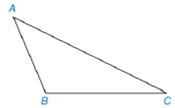Chapter 7.1, Problem 7EElementary Geometry For College St...

7th Edition
Alexander + 2 others
ISBN: 9781337614085

Solutions

Chapter
SectionElementary Geometry For College St...

7th Edition
Alexander + 2 others
ISBN: 9781337614085
Textbook Problem

Note: Exercises preceded by an asterisk are of a more challenging nature.In Exercises 3 to 8, use the drawing provided.Given: Obtuse ∆ A B C Construct: The altitude from A to B C -

To determine

To construct:

The altitude from A to BC-

Explanation

Perpendicular bisector of a triangle is a line passing through the midpoint of the particular side of the triangle.

Calculation:

The obtuse ABC is given

To construct a bisector we follow the below procedure.

Step 1: Extend the line BC.

Step 2: Draw a line from the point A perpendicular to the extension of the line BC

Still sussing out bartleby?

Check out a sample textbook solution.

See a sample solution

The Solution to Your Study Problems

Bartleby provides explanations to thousands of textbook problems written by our experts, many with advanced degrees!

Get Started

Polynomials Find the sum, difference, or product. 15. (12x 7) (5x 12)

Precalculus: Mathematics for Calculus (Standalone Book)

In Exercises 1-8, determine which of the matrices are regular. 

Finite Mathematics for the Managerial, Life, and Social Sciences

Using a binomial series, the Maclaurin series for is:

Study Guide for Stewart's Multivariable Calculus, 8th